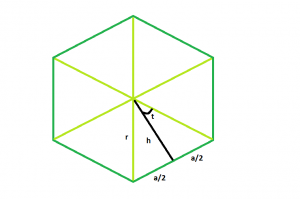# Apothem of a n-sided regular polygon

Given here the side length a of a regular n-sided polygon, the task is to find the length of its Apothem.
Apothem is the line drawn from the center of the polygon that is perpendicular to one of its sides.
Examples:

```Input a = 9, n = 6
Output: 7.79424

Input: a = 8, n = 7
Output: 8.30609
```## Recommended: Please try your approach on {IDE} first, before moving on to the solution.

Approach:

In the figure, we see the polygon can be divided into n equal triangles.
Looking into one of the triangles, we see the whole angle at the centre can be divided into = 360/n
So, angle t = 180/n
now, tan t = a/2h
So, h = a/(2*tan t)
here, h is the apothem,
so, apothem = a/(2*tan(180/n))

Below is the implementation of the above approach.

## C++

 `// C++ Program to find the apothem ` `// of a regular polygon with given side length ` `#include ` `using` `namespace` `std; ` ` `  `// Function to find the apothem ` `// of a regular polygon ` `float` `polyapothem(``float` `n, ``float` `a) ` `{ ` ` `  `    ``// Side and side length cannot be negative ` `    ``if` `(a < 0 && n < 0) ` `        ``return` `-1; ` ` `  `    ``// Degree converted to radians ` `    ``return` `a / (2 * ``tan``((180 / n) * 3.14159 / 180)); ` `} ` ` `  `// Driver code ` `int` `main() ` `{ ` `    ``float` `a = 9, n = 6; ` `    ``cout << polyapothem(n, a) << endl; ` ` `  `    ``return` `0; ` `} `

## Java

 `// Java Program to find the apothem of a  ` `// regular polygon with given side length ` `import` `java.util.*; ` ` `  `class` `GFG ` `{ ` ` `  `    ``// Function to find the apothem ` `    ``// of a regular polygon ` `    ``double` `polyapothem(``double` `n, ``double` `a) ` `    ``{ ` ` `  `        ``// Side and side length cannot be negative ` `        ``if` `(a < ``0` `&& n < ``0``) ` `            ``return` `-``1``; ` ` `  `        ``// Degree converted to radians ` `        ``return` `(a / (``2` `* java.lang.Math.tan((``180` `/ n) ` `                ``* ``3.14159` `/ ``180``))); ` `    ``} ` ` `  `// Driver code ` `public` `static` `void` `main(String args[]) ` `{ ` `    ``double` `a = ``9``, n = ``6``; ` `    ``GFG g=``new` `GFG(); ` `    ``System.out.println(g.polyapothem(n, a)); ` `} ` ` `  `} ` `//This code is contributed by Shivi_Aggarwal `

## Python3

 `# Python 3 Program to find the apothem ` `# of a regular polygon with given side ` `# length ` `from` `math ``import` `tan ` ` `  `# Function to find the apothem ` `# of a regular polygon ` `def` `polyapothem(n, a): ` `     `  `    ``# Side and side length cannot be negative ` `    ``if` `(a < ``0` `and` `n < ``0``): ` `        ``return` `-``1` ` `  `    ``# Degree converted to radians ` `    ``return` `a ``/` `(``2` `*` `tan((``180` `/` `n) ``*`  `                   ``3.14159` `/` `180``)) ` ` `  `# Driver code ` `if` `__name__ ``=``=` `'__main__'``: ` `    ``a ``=` `9` `    ``n ``=` `6` `    ``print``(``'{0:.6}'``.``format``(polyapothem(n, a))) ` `     `  `# This code is contributed by ` `# Sahil_Shelangia `

## C#

 `// C# Program to find the apothem of a  ` `// regular polygon with given side length  ` `using` `System; ` ` `  `class` `GFG  ` `{  ` ` `  `// Function to find the apothem  ` `// of a regular polygon  ` `static` `double` `polyapothem(``double` `n,  ` `                          ``double` `a)  ` `{  ` ` `  `    ``// Side and side length cannot ` `    ``// be negative  ` `    ``if` `(a < 0 && n < 0)  ` `        ``return` `-1;  ` ` `  `    ``// Degree converted to radians  ` `    ``return` `(a / (2 * Math.Tan((180 / n) *  ` `                       ``3.14159 / 180)));  ` `}  ` ` `  `// Driver code  ` `public` `static` `void` `Main()  ` `{  ` `    ``double` `a = 9, n = 6;  ` `    ``Console.WriteLine(Math.Round(polyapothem(n, a), 4));  ` `}  ` `}  ` ` `  `// This code is contributed by Ryuga `

## PHP

 ` `

Output:

```7.79424
```

My Personal Notes arrow_drop_upCheck out this Author's contributed articles.

If you like GeeksforGeeks and would like to contribute, you can also write an article using contribute.geeksforgeeks.org or mail your article to contribute@geeksforgeeks.org. See your article appearing on the GeeksforGeeks main page and help other Geeks.

Please Improve this article if you find anything incorrect by clicking on the "Improve Article" button below.

Article Tags :
Practice Tags :

Be the First to upvote.

Please write to us at contribute@geeksforgeeks.org to report any issue with the above content.